Molecular orbital diagrams provide qualitative information about the structure and stability of the electrons in a molecule. This article explains how to create molecular orbital diagrams in LaTeX by means of the package MOdiagram. For information about the more traditional molecular structure diagrams see our documentation about chemistry formulae.

## Introduction

Molecular diagrams are created using the environment MOdiagram. Below you can see the simplest working example:

\documentclass{article}
\usepackage[utf8]{inputenc}

\usepackage{modiagram}

\begin{document}

\begin{MOdiagram}
\atom{left}{1s, 2s, 2p}
\end{MOdiagram}

\end{document}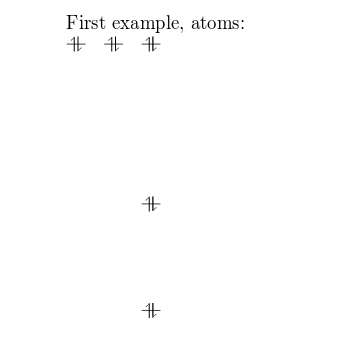First, the package MOdiagrams is imported by

\usepackage{modiagram}


The basic command to draw MO diagrams is \atom. This command has two parameter in the example:

• left. The alignment of the atom.
• 1s, 2s, 2p Are the energy sub-levels to be drawn. These can be further customized as you will learn in the next section.

## Atoms

You can pass some extra information about the atomic orbitals to the command presented in the introductory example.

\begin{MOdiagram}
\atom{right}{
1s = { 0; pair} ,
2s = { 1; pair} ,
2p = {1.5; up, down }
}

\atom{left}{
1s = { 0; pair} ,
2s = { 1; pair} ,
2p = {1.5; up, down }
}
\end{MOdiagram}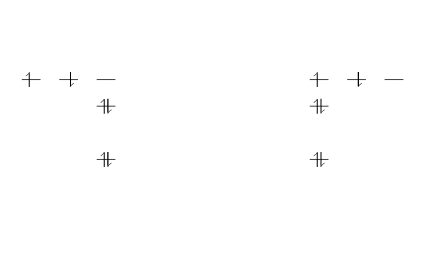In this example, two identical atoms are drawn, left and right-aligned respectively. The second argument in the command \atom contains some extra information. The generic syntax to create atoms is:

1s = {energy; specifications}


instead of 1s other possible values are 2s and 2p

• energy. Is the energy level, this number determines the vertical spacing in the diagram. If omitted is set to 0.
• specifications. A comma-separated list of the spins of the electrons contained in each orbital. The possible values are up, down, pair and empty (only the semicolon is typed) for an empty orbital. If omitted is set to pair.

For a better understanding of this syntax, below is a description of the commands in the previous example:

• 1s = { 0; pair}. The sub-level 1s is in the 0 energy level, the orbital contains two (paired) electrons.
• 2s = { 1; pair}. The sub-level 2s drawn in the 1 energy level, there are two electrons in this orbital.
• 2p = {1.5; up, down}. The sub-level 2p drawn in the energy level 1.5, i.e. in the diagram the vertical spacing is set to 1.5; this sub-energy level has two electrons: one with spin up in the first orbital and another with spin down in the second orbital.

The same commands are repeated for the second atom on the right.

## Molecules

The syntax for molecules is very similar to that of the \atom command presented in the previous section. The energy sub-levels 1s, 2s and 2p become 1sMO, 2sMO and 2pMO respectively.

\begin{MOdiagram}
\atom{left}{1s}
\atom{right}{1s={;up}}
\molecule{
1sMO={0.75;pair,up}
}
\end{MOdiagram}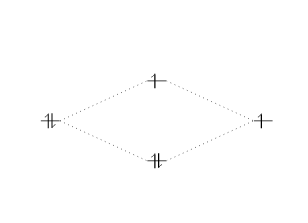The syntax for \molecule takes two parameters just as the command \atom, but the orbital specifications are slightly different.

1sMO={0.75;pair,up}

• 0.75 is now the ratio (energy gain)/(energy loss).
• pair, up are the spins of the electrons in the bonding and anti-bonding molecular orbitals, respectively.

Below you can see a more elaborate example to help understanding the syntax.

\begin{MOdiagram}
\atom{left}{
1s, 2s, 2p = {;pair,up,up}
}
\atom{right}{
1s, 2s, 2p = {;pair,up,up}
}
\molecule{
1sMO, 2sMO, 2pMO = {;pair,pair,pair,up,up}
}
\end{MOdiagram}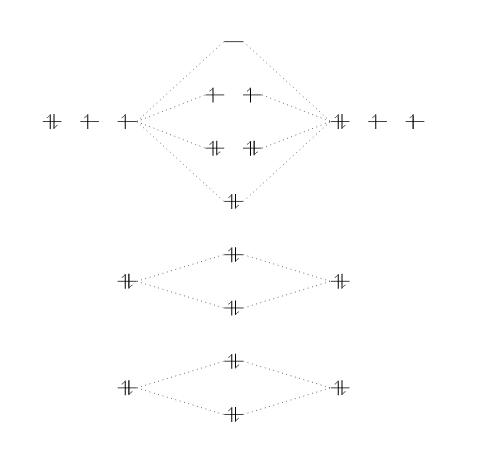Three atoms are set on each side of the diagram and the corresponding molecule in the middle.

## Naming scheme

There is no straightforward way to set captions and names for elements in a MO diagram, but since this package is based on TikZ you can use tikz commands within the MOdiagram environment:

\begin{MOdiagram}
\atom{left}{1s}
\atom{right}{1s={;up}}
\molecule{
1sMO={;pair,up}
}
\draw[<-,shorten <=8pt,shorten >=15pt,blue]
(1sigma*) --++(2,1) node {anti-bonding MO};
\end{MOdiagram}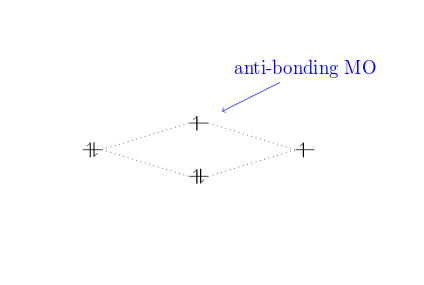Notice that the name of the anti-bonding orbital 1sigma* can be used for relative positions. Other labels available are:

• 1sigma
• 2sigma
• 2pisigma
• 2piy
• 2piz

and the corresponding starred versions.